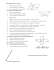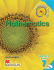# MAT.04.ER.2.000MD.A.049 Claim 2 Grade 4 Mathematics Sample ER Item Claim 2

## Transcription

MAT.04.ER.2.000MD.A.049 Claim 2 Grade 4 Mathematics Sample ER Item Claim 2
```Grade 4 Mathematics Sample ER Item Claim 2
MAT.04.ER.2.000MD.A.049 Claim 2
Sample Item Id:
Primary Claim:
Secondary Claim(S):
Primary Content Domain:
Secondary Content Domain(S):
Assessment Target(S):
MAT.04.ER.2.000MD.A.049
04
Claim 2: Problem Solving
Students can solve a range of well-posed problems in
pure and applied mathematics, making productive use
of knowledge and problem-solving strategies.
Claim 1: Concepts and Procedures
Students can explain and apply mathematical concepts
and carry out mathematical procedures with precision
and fluency.
Measurement and Data
2 A: Apply mathematics to solve well-posed problems
arising in everyday life, society, and the workplace.
2 B: Select and use appropriate tools strategically.
Standard(S):
Mathematical Practice(S):
DOK:
Item Type:
Score Points:
Difficulty:
Key:
Stimulus/Source:
Target-Specific Attributes
(E.G., Accessibility Issues):
Notes:
1 K: Geometric measurement: understand concepts of
angle and measure angles.
4.MD.6, 4.MD.7
1, 2, 4, 5, 6
2
ER
3
M
See Sample Top-Score Response.
Multi-part item; requires use of online protractor. First
two response boxes allow up to 3 numeric entries and
no degree symbol.
Version 1.0
Grade 4 Mathematics Sample ER Item Claim 2
Look at the figure below.
Use the online protractor to measure angle KHJ in degrees.
Click in the box and then type the measure of angle KHJ.
degrees
The measure of angle GHJ is 90 degrees. Use the measure of
angle GHJ to find the measure of angle GHK, in degrees.
degrees
In the space below, use pictures, numbers, and/or words to
show how you found the measure of angle GHK.
Version 1.0
Grade 4 Mathematics Sample ER Item Claim 2
Sample Top-Score Response:
25
65
I know that the measure of angles KHJ and GHK should add up to be the same measure as
angle GHJ. If angle GHJ has a measure of 90°, then the other two angles together have to
have that measure. I subtracted 25° from 90° to get 65°. I could also use these equations:
90 – 25 = 65 or 65 + 25 = 90.
Scoring Rubric:
Responses to this item will receive 0-3 points, based on the following:
3 points: Student has thorough understanding of measuring angles in whole-number
degrees using a protractor and recognizing angle measure as additive. The
student completes the following:
• Writes 25 for the measure of angle KHJ (+/- 2 degrees).
• Writes 65 for the measure of angle GHJ (+/- 2 degrees).
• Writes a thorough and correct explanation of reasoning, as shown in
Sample Top-Score Response.
2 points: Student has partial understanding of measuring angles in whole-number degrees
using a protractor and recognizing angle measure as additive. The student
completes the first 2 bullets, but provides an explanation of reasoning that is
incomplete or incorrect. OR The student makes a mistake in the first bullet,
writes a correct measure for angle GHJ incorporating this incorrect angle
measure, and provides a thorough and correct explanation of reasoning.
1 point: The student has limited understanding of measuring angles in whole-number
degrees using a protractor and recognizing angle measure as additive. The
student correctly completes only the first bullet.
0 points: Shows little or no understanding of measuring angles in whole-number degrees
using a protractor and recognizing angle measure as additive.
Version 1.0
```

### Build an Angle Setting Jig to Sharpening Plane Irons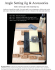### If ABC is obtuse, what are the restrictions on x? ∠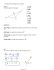### installation of an angle board * * * thermopian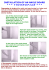### Document 6592969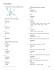### Nanocomp Pattern generators, PDF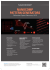### Class V-Unit 10 - International Indian School Jeddah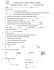### Smart Tool - Benchmark Precision Instruments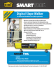### 12 Solving Percents Using Proportions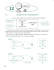### MATHS WORKSHEET NAME GRADE 6 Date I. Evaluate the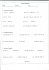### Measuring and Classifying Angles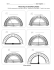### Reflection (from Mulan) Rose is Rose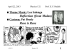### Adjustable Angle Composite Sheeter Options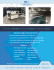### Reteach Sec 1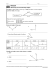### STUDY GUIDE for Unit 1 Test Geometry 1. Z?